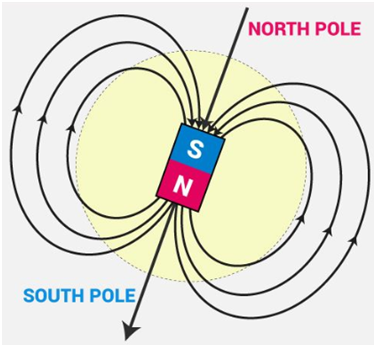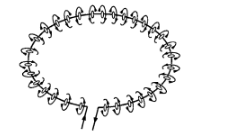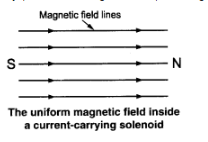• +91 9971497814
• info@interviewmaterial.com

# Chapter 13- Magnetic Effects of Electric Current Interview Questions Answers

### Related Subjects

Question 1 : Why does a compass needle get deflected when brought near a bar magnet?

Answer 1 : The compass needle is a small magnet. When the compass needle is brought close to a bar magnet, the magnetic field lines of the compass needle interact with the magnetic field lines of bar magnet, which causes the compass needle to deflect.

Question 2 : Draw magnetic field lines around a bar magnet.

Answer 2 : Magnetic field lines of a bar magnet emerge from the North Pole and terminate at the South Pole as shown in the figure below.Question 3 : List the properties of magnetic field lines.

The properties of magnetic field lines are as follows:
Magnetic field lines do not intersect with each other.
They emerge from the North Pole and terminate at the South Pole.
Inside the magnet, the direction of the field lines is from the South Pole to the North Pole.

Question 4 : Why don’t two magnetic field lines intersect each other?

If two magnetic field lines intersect then at the point of intersection the compass needle shows two different directions, which is not possible, hence they do not intersect with each other.

Question 5 : Consider a circular loop of wire lying in the plane of the table. Let the current pass through the loop clockwise. Apply the right-hand rule to find out the direction of the magnetic field inside and outside the loop.

Answer 5 :As shown in figure alongside, each section of wire produces its concentric set of lines of force. By applying right hand thumb rule, we find that all the sections produce magnetic field downwards at all points inside the loop while at the outside points, the field is directed upwards. Therefore, the magnetic field acts normally into the plane of the paper at the points inside the loop and normally out of the plane of paper at points outside the loop.

Question 6 : The magnetic field in a given region is uniform. Draw a diagram to represent it.

A uniform magnetic field in a region is represented by drawing parallel straight lines, ail pointing in the same direction.
For example, the uniform magnetic field which exists inside a current-carrying solenoid can be represented by parallel straight lines pointing from its S-pole to N-pole (as shown in figure).Question 7 :
Choose the correct option.
The magnetic field inside a long straight solenoid-carrying current

1. is zero.
2. decreases as we move towards its end.
3. increases as we move towards its end.
4. is the same at all points.

d. is the same at all points
The magnetic field inside a long straight current carrying solenoid is uniform therefore it is the same at all points.

Question 8 : Which of the following property of a proton can change while it moves freely in a magnetic field?

(There may be more than one correct answer.)
1. Mass                            2. Speed
3. Velocity                         4. Momentum

(c) and (d)
When a proton enters the region of magnetic field, it experiences magnetic force. Due to which the path of the proton becomes circular. As a result, the velocity and the momentum change.

Question 9 : In Activity 13.7, how do we think the displacement of rod AB will be affected if

Answer 9 : (i) current in rod AB is increased;

(ii) a stronger horse-shoe magnet is used; and
(iii) length of the rod AB is increased?

A current carrying conductor when placed in a magnetic field experiences force. The magnitude of this force will increase with the increase in the amount of current, length of conductor and the strength of the magnetic field. Hence, the strength of the magnetic force exerted on the rod AB and its displacement will increase if
1. The current in rod AB is increased
2. Stronger horse shoe magnet is used
3. When the length of the rod AB increases

Question 10 : A positively-charged particle (alpha-particle) projected towards the west is deflected towards north by a magnetic field. The direction of magnetic field is

1. towards south                               2. towards east
3. downward                                     4. upward

The direction of the magnetic field can be determined using the Fleming’s Left hand rule. According to the rule, if we arrange our thumb, forefinger and the middle finger of the left hand right perpendicular to each other, then the thumb points towards the direction of the magnetic force, the middle finger the direction of current and the forefinger the direction of magnetic field. Since the direction of positively charged particle is towards west, the direction of the current will also be towards the west. The direction of the magnetic force is towards the north, hence the direction of magnetic field will be upward according to Fleming’s Left hand rule.

Todays Deals### Chapter 13- Magnetic Effects of Electric Current Contributorskrishan

Name:
Email:

# Latest News# 9000 interview questions in different categories